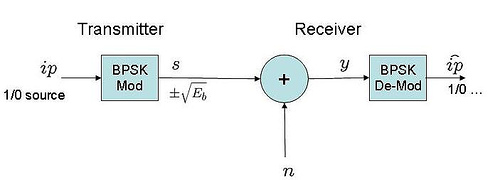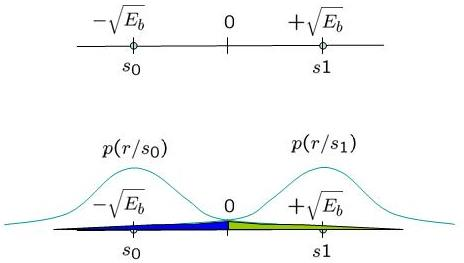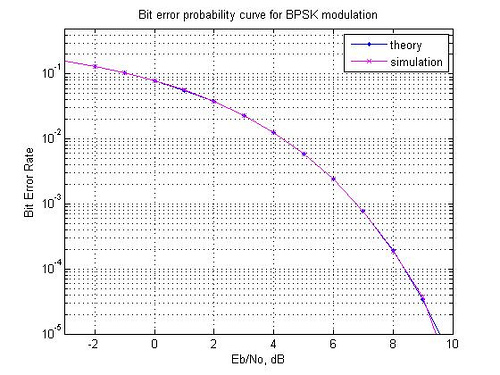- DSP log - http://www.dsplog.com -

Bit Error Rate (BER) for BPSK modulation

Posted By Krishna Sankar On August 5, 2007 @ 8:17 pm In Modulation | 473 Comments

In this post, we will derive the theoretical equation for bit error rate (BER) with Binary Phase Shift Keying (BPSK) modulation scheme in Additive White Gaussian Noise (AWGN) channel. The BER results obtained using Matlab/Octave simulation scripts show good agreement with the derived theoretical results.

With Binary Phase Shift Keying (BPSK), the binary digits 1 and 0 maybe represented by the analog levels and respectively. The system model is as shown in the Figure below.Figure: Simplified block diagram with BPSK transmitter-receiver

## Channel Model

The transmitted waveform gets corrupted by noise , typically referred to as Additive White Gaussian Noise (AWGN).

White : The spectrum of the noise if flat for all frequencies.

Gaussian : The values of the noise follows the Gaussian probability distribution function, with and .

## Computing the probability of error

Using the derivation provided in Section 5.2.1 of [COMM-PROAKIS]  as reference:

when bit 1 is transmitted and

when bit 0 is transmitted.

The conditional probability distribution function (PDF) of for the two cases are:

.Figure: Conditional probability density function with BPSK modulation

Assuming that and are equally probable i.e. , the threshold 0 forms the optimal decision boundary.

• if the received signal is is greater than 0, then the receiver assumes was transmitted.
• if the received signal is is less than or equal to 0, then the receiver assumes was transmitted.

i.e.

and

.

### Probability of error given was transmitted

With this threshold, the probability of error given is transmitted is (the area in blue region):

,

where,

is  the complementary error function.

### Probability of error given was transmitted

Similarly the probability of error given is transmitted is (the area in green region):

.

### Total probability of bit error

.

Given that we assumed that and are equally probable i.e. , the bit error probability is,

.

## Simulation model

Matlab/Octave source code for computing the bit error rate with BPSK modulation from theory and simulation. The code performs the following:

(a) Generation of random BPSK modulated symbols +1′s and -1′s

(b) Passing them through Additive White Gaussian Noise channel

(c) Demodulation of the received symbol based on the location in the constellation

(d) Counting the number of errors

(e) Repeating the same for multiple Eb/No value.Figure: Bit error rate (BER) curve for BPSK modulation – theory, simulation

## Reference

[DIGITAL COMMUNICATION: PROAKIS]Digital Communications by John Proakis 

URL to article: http://www.dsplog.com/2007/08/05/bit-error-probability-for-bpsk-modulation/

URLs in this post: